# Private Equity multiples

Private Equity multiples are calculated by (qualified) investors to evaluate the performance of private equity funds. Very often, private equity funds exhibit a so-called J-curve effect. This means that the fund initially posts negative returns because the PE firm is investing money. After a few years, however, the returns turn positive as the portfolio companies are sold.

On this page, we discuss the most common PE multiples and calculate the multiples using a private equity fee calculation example. A spreadsheet that implements the multiples is available for download below.

## Private equity multiples definitions

There are four Private Equity (PE) ratios we should consider when evaluating a PE firm. The first one is the paid-in capital (PIC). Paid-in capital equals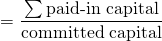Next, there is distributed to paid-in capital (DPI). DPI measures the realized return of the limited partner. The formula for DPI is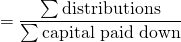Residual Value to Paid-in Capital (RVPI) measures the unrealized return. It equals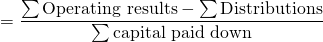Finally, TVPI or total value to paid-in capital is the sum of RVPI and DPI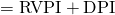## Private Equity Multiples calculation example

Let’s apply the above formulae using an actual numerical example. To calculate the above statistics, it is best that we start from a table that already calculates all the necessary data. The best way, in fact, is to first calculate the fees and carried interest. Once we have those, we can calculate the multiples we need. The table below illustrates this approach.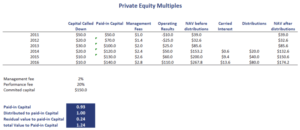## Summary

We discussed the calculation of Private Equity ratios. These multiples allow investors to compare different companies as well as determine the stage the PE firm is in. This is important, considering that PE firms tend to exhibit a J-curve effect.

### Private Equity ratios

Want to have an implementation in Excel? Download the Excel file: Private Equity ratios